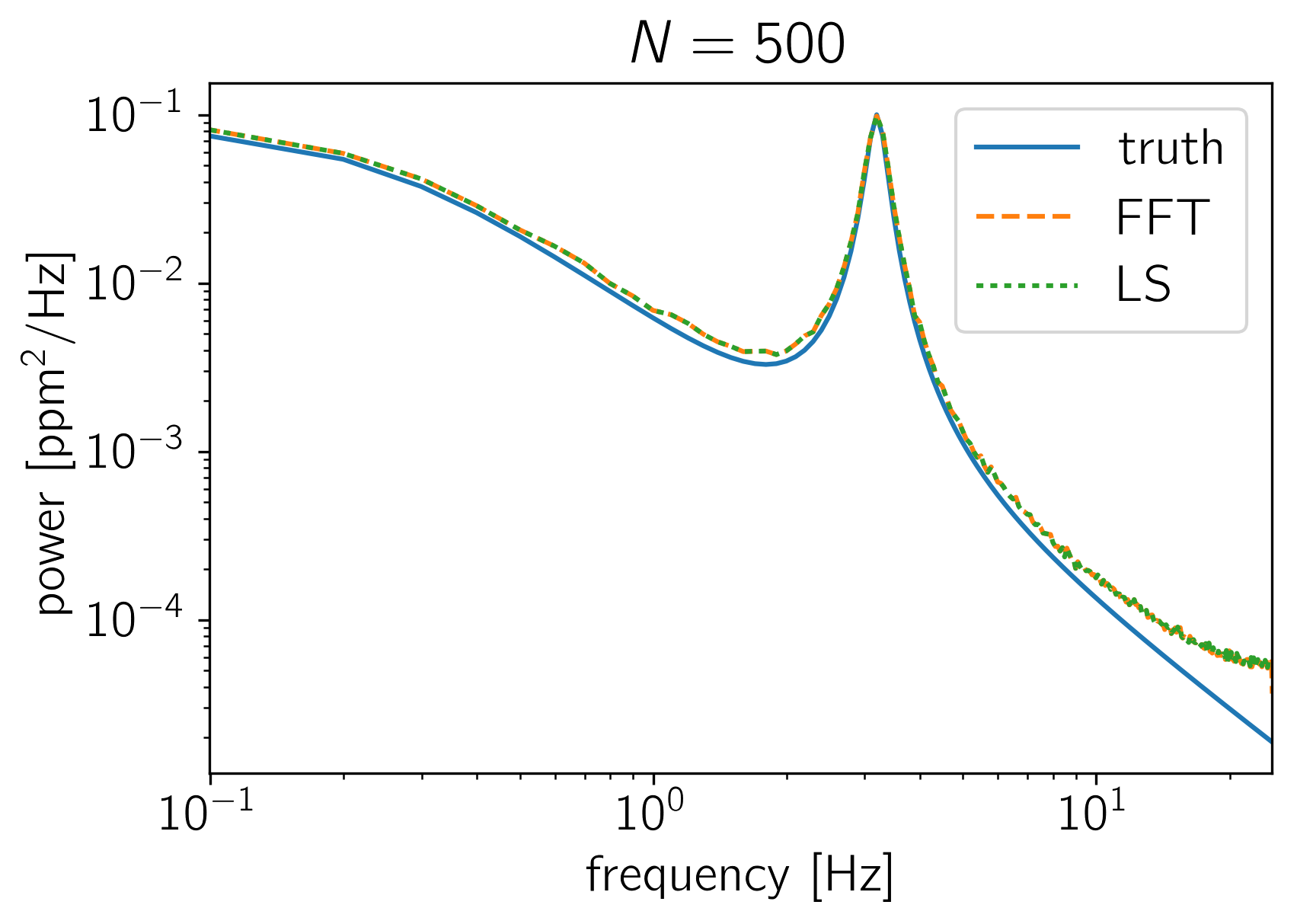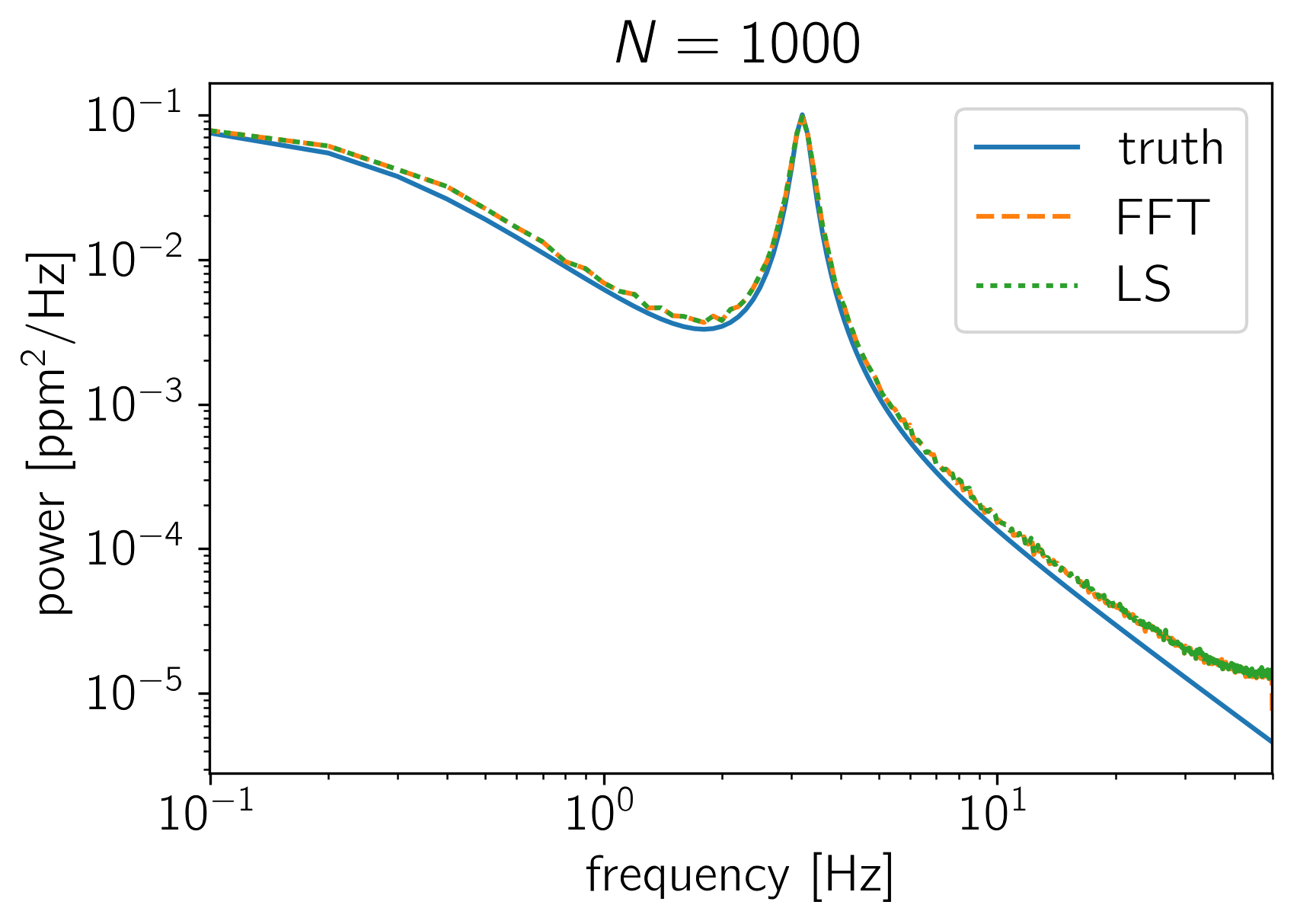Note

This tutorial was generated from an IPython notebook that can be downloaded here.

# PSD Normalization¶

The crux of many time series analysis problems is the question of where all the factors of $$N$$ and $$2\,\pi$$ enter. In this tutorial, we’ll look at how the PSD returned by celerite should be compared to an estimate made using NumPy’s FFT library or to an estimate made using a Lomb-Scargle periodogram. To make this comparison, we’ll sample many realizations from a celerite GP and compute the empirical power spectrum using the standard methods and compare this (numerically) to the true power spectrum as defined by celerite.

To start, here’s a function that simulates $$K$$ random datasets with $$N$$ data points from a celerite model and computes the mean FFT and Lomb-Scargle estimators of the power spectrum.

import numpy as np
import matplotlib.pyplot as plt
from astropy.stats import LombScargle

import celerite
from celerite import terms

def simulate_and_compute_psds(N, K=1500):
# Set up a simple celerite model
kernel = terms.RealTerm(0.1, 0.5) + terms.ComplexTerm(0.5, 0.05, 3.0)
gp = celerite.GP(kernel)

# Simulate K datasets with N points
t = np.linspace(0, 10, N)
gp.compute(t)
np.random.seed(42)
y = gp.sample(size=K)

# Compute the FFT based power spectrum estimates
f = np.fft.rfftfreq(len(t), t - t)
fft = np.array(list(map(np.fft.rfft, y)))
fft *= np.conj(fft)

# >>> To get the FFT based PSD in the correct units, normalize by N^2 <<<
power_fft = fft.real / N**2

# Compute the LS based power spectrum estimates
power_ls = []
for y0 in y:
model = LombScargle(t, y0)
power_ls.append(model.power(f[1:-1], method="fast", normalization="psd"))
power_ls = np.array(power_ls)

# >>> To get the LS based PSD in the correct units, normalize by N <<<
power_ls /= N

# Compute the true power spectrum
# NOTE: the 2*pi enters because celerite computes the PSD in _angular_ frequency
power_true = kernel.get_psd(2*np.pi*f)

# >>> To get the true PSD in units of physical frequency, normalize by 2*pi <<<
power_true /= 2*np.pi

# Let's plot the estimates of the PSD
plt.figure()
plt.plot(f, power_true, label="truth")
plt.plot(f, np.median(power_fft, axis=0), "--", label="FFT")
plt.plot(f[1:-1], np.median(power_ls, axis=0), ":", label="LS")
plt.yscale("log")
plt.xscale("log")
plt.xlim(f.min(), f.max())
plt.ylabel("power [$\mathrm{ppm}^2/\mathrm{Hz}$]")
plt.xlabel("frequency [Hz]")
plt.title("$N = {0}$".format(N))
plt.legend()

simulate_and_compute_psds(500)
simulate_and_compute_psds(1000)

(1500, 500)
(1500, 1000)